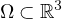Electron. J. Differential Equations, Vol. 2022 (2022), No. 07, pp. 1-18.

### Asymptotic behavior of solutions to 3D Kelvin-Voigt-Brinkman-Forchheimer equations with unbounded delays Le Thi Thuy

Abstract:
In this article we consider a 3D Kelvin Voigt Brinkman Forchheimer equations involving unbounded delays in a bounded domain. First, we show the existence and uniqueness of weak solutions by using the Galerkin approximations method and the energy method. Second, we prove the existence and uniqueness of stationary solutions by employing the Brouwer fixed point theorem. Finally, we study the stability of stationary solutions via the direct classical approach and the construction of a Lyapunov function. We also give a sufficient condition for the polynomial stability of the stationary solution for a special case of unbounded variable delay.

Submitted November 3, 2020. Published January 17, 2022.
Math Subject Classifications: 35B41, 35K65, 35D05
Key Words: Kelvin-Voigt-Brinkman-Forchheimer equation; delay equation; stationary solution; local stability; asymptotically stable; polynomial stable.

Show me the PDF file (364 KB), TEX file for this article.Le Thi Thuy Department of Mathematics Electric Power University 235, Hoang Quoc Viet Bac Tu Liem, Hanoi, Vietnam email: thuylt@epu.edu.vn, thuylephuong@gmail.com